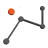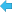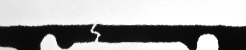Back to Adaptive Vision Studio website# PointAlongPath

Transforms a point to a coordinate system in which the 'axis' path is vertical or horizontal.

Name Type DescriptioninPoint Point2D Input pointinAxis Path Input axis pathinAxisType Axis Type of axis the input axis path is parallel toinAxisCoordinate Real Coordinate of the axis pathinInverse Bool Switches to the inverse operationoutPoint Point2D Transformed point

### Applications

Usually used to revert an ImageAlongPath transformation.

### Description

This operation transforms a point - inPoint - between two coordinate systems: the one linked with 'axis' path inAxis and the original one. Direction of this conversion is based on inInverse value:

• if it is true, inPoint is converted to original coordinate system
• if it is false, inPoint is converted from original coordinate system

Coordinate system linked with inAxis is some transformation of the original one, satisfying these conditions:

• 'axis' path is represented as a straight line
• 'axis' path is horizontal if inAxisType is set to X or vertical if inAxisType is set to Y
• if inAxisType is set to X, the Y coordinate of 'axis' path is equal to inAxisCoordinate. If inAxisType is set to Y, the X coordinate of 'axis' path is equal to inAxisCoordinate

### Hints

• If this filter is used to reverse ImageAlongPath transformation you should set inAxis and inAxisType to the same values as corresponding ones in ImageAlongPath. inAxisCoordinate should be set to a half of ImageAlongPath.inScanWidth.

### ExamplesImageAlongPath performed on the sample image with inAxisType = X, inScanWidth = 50 and path marked with blue. Point marked with blue cross on the output image was calculated by ScanSingleEdge. Original point (marked with orange cross) was calculated using PointAlongPath with inAxisType = X, inAxisCoordinate = 25, inInverse = true and position of detected point. ImageAlongPath and PointAlongPath used the same path for transformations.

### Errors

This filter can throw an exception to report error. Read how to deal with errors in Error Handling.

List of possible exceptions:

Error type Description
DomainError Empty path on input in PointAlongPath.

### Complexity Level

This filter is available on Advanced Complexity Level.